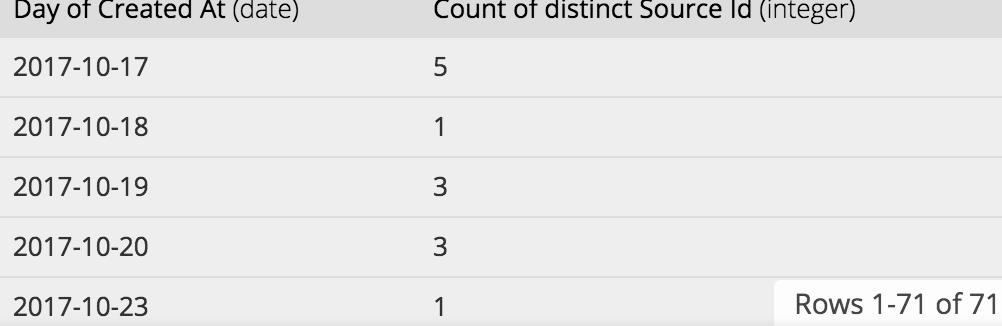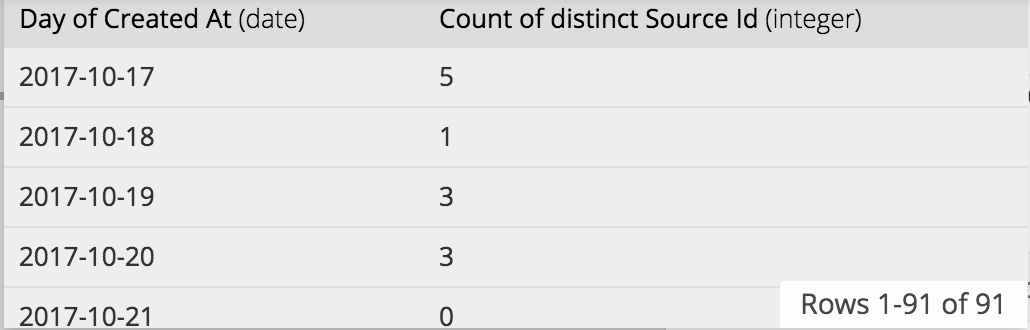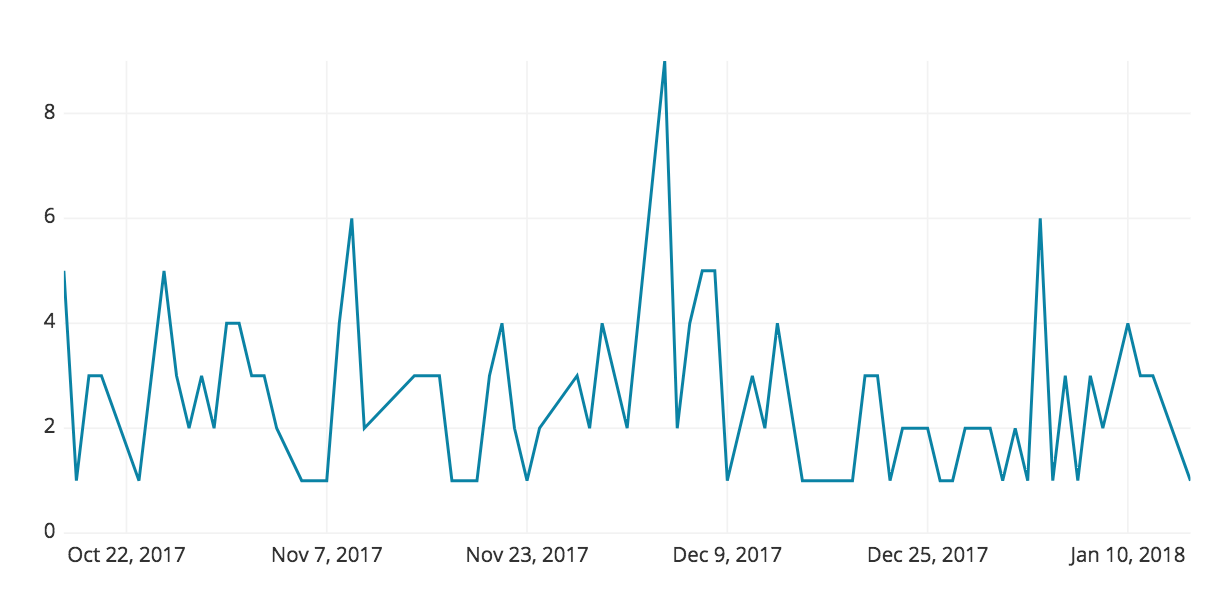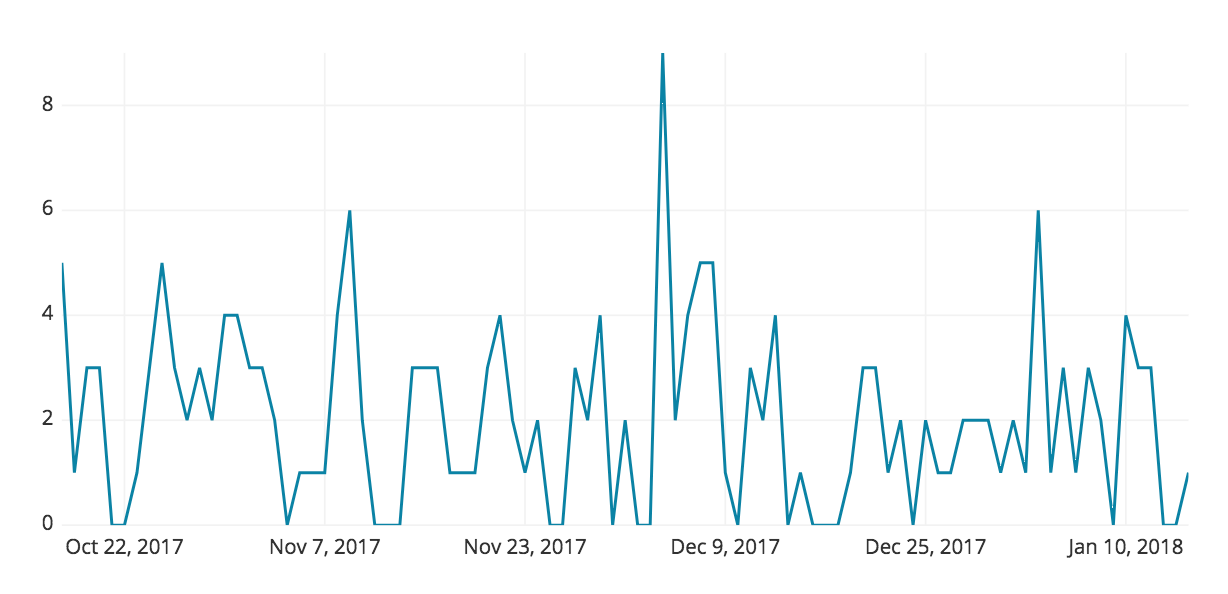# How to Generate Series (Time) in Redshift

###### Data TutorialAmazon Redshift

Charts/Tables that include time series are great for visualizing data over time and quickly recognizing trends. But incomplete time series can cause gaps and alter results that can be misleading. Take for example, the time series below:On the left, the series looks like it always has a consistent count with no dips to zero. On the right, after adding in the missing dates, the series does drop to zero showing a more drastic variation between each day.

An easy way of fixing incomplete time series is to join it with a complete list. You can do this easily with PostgreSQL using the generate_series function. In the example below, you can use the generate_series function with any PostgreSQL database to generate a continuous time series:

``````select *
from generate_series('2018-01-01'::TIMESTAMP, '2019-01-01'::TIMESTAMP, '1 day')
``````

Which produces:You can use the generate_series with any two timestamps including PostgreSQL’s built in function that returns timestamps (ex: CURRENT_TIMESTAMP) or Chartio’s built in relative dates (ex: {TODAY}::TIMESTAMP). For more on generate series functions in PostgreSQL: Generate Series in PostgreSQL

## How to Generate Series Without the generate_series Function

But not all databases have a generate_series function. For databases that don’t natively support generate_series, using alternative methods can still accomplish the same result.

An easy alternative that can work with any database is using a continuous list of numbers starting at zero that can be converted into a date. You can either create the table containing the list of numbers or utilize an existing column in a table if that table contains a sequential row id column with no deleted rows. You can then convert each number into a date by subtracting the current date with the numbers column to build a continuous list of dates.

For these examples, n is the number column that contains a continuous list of numbers and numbers_table is the table that contains the n column.

Amazon Redshift

``````SELECT (getdate()::date - n)::date FROM numbers_table
``````

MySQL

``````SELECT date_sub(CURDATE()), interval n DAY) FROM numbers_table
``````

``````SELECT date_sub(CURRENT_DATE, interval n DAY) FROM numbers_table
``````

If you don’t have an existing numbers column or table, you can create one.

FOR MySQL DATABASE In MySQL, you can use variables to generate a numbers column using a table that has at least as many rows as the number of dates you want to generate and a limit to determine how many dates you want to generate.

``````    set @n:=CURDATE() + interval 1 day;
select (select @n:= @n - interval 1 day) number_column
from any_table limit 100
``````

FOR DATABASE WITH WINDOW FUNCTIONS Amazon Redshift, Google BigQuery

Another option for generating a continuous series is to use a window function. You can use a window function to build one using a table that has at least as many rows as the number of dates you want to generate and a limit to determine how many dates you want to generate.

Amazon Redshift

``````    select (getdate()::date - row_number() over (order by true))::date as n
from any_table limit 100
``````

``````    SELECT date_sub(CURRENT_DATE, interval ROW_NUMBER() OVER () DAY) FROM any_table limit 100